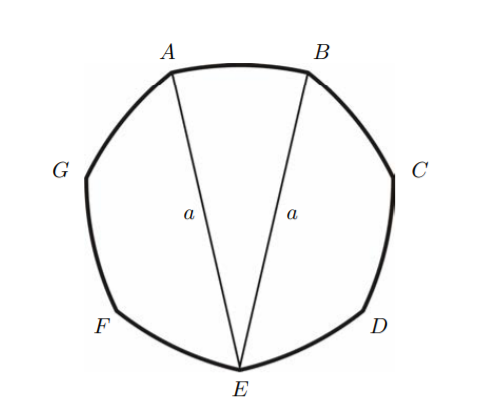墨滴Opsimath

2021/09/18  阅读：19  主题：默认主题

# STEP 6

The question today comes from 1987 Specimen Paper I. The original question is shown below.The diagram shows a British 50 pence coin. The seven arcs are of equal length and each arc is formed from the circle of radius having its centre at the vertex diametrically opposite the mid-point of the arc. Show that the area of the coin is

One thing special about this figure (the Reuleaux polygon) is that it has constant diameter; it can roll between two parallel lines without losing contact with either, which is kinda difficult to prove. The question itself, though, is not hard.

Solution. Label the centre of the circle as and connect .

In the figure, the point is equidistant from each of three vertices and . The plan is to find the area of the sector by calculating the area of and subtracting the areas of the two congruent isosceles triangles and . The required area is 7 times this.

First, we need angle . We know that and hence . Finally, .

Now, is a sector of a circle of radius , so its area is . The area of triangle is , i.e., . The area of the coin is therefore which reduces to the given answer.

Extension. Consider the area of an -sided coin similar to this and check that as , the formula gives the correct answer for a circle of diameter .Opsimath

2021/09/18  阅读：19  主题：默认主题